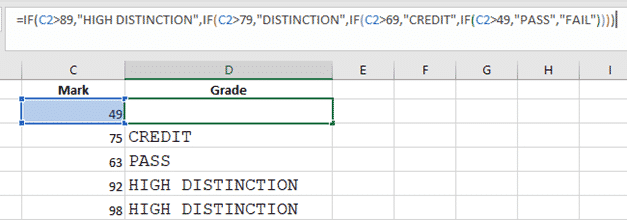# More Excel alternatives to nested IF statements

Nested IF statements can be hard to understand and manage but there are alternatives which can be easier to handle, fix and change.

We’ll start with the relatively simple example we used for demonstrating IF and nested IF.

`=IF(C2>89,"HIGH DISTINCTION",IF(C2>79,"DISTINCTION",IF(C2>69,"CREDIT",IF(C2>49,"PASS","FAIL"))))`This example has only five levels of nesting, we’ve seen nested IF’s with many, many more than that.  Those long formulas can be very hard to understand (especially if you’re new to the worksheet) and debug (one missing or extra comma is enough).

Happily, there are alternatives which return the same result but are a lot easier to understand, change and fix when they go wrong.

We’ve already mentioned IFS, the alternative to nested IF’s but only available in Excel 365 and Excel 2019.

## VLookup / XLookup

This alternative has a lookup table of values and results plus a formula to lookup the result.

Start by making a table of values and results.Then use either Vlookup() or Xlookup() to lookup marks and return a grade.

Xlookup() is the new Excel 365 function.  It’s a far better and easier option than Vlookup() or Hlookup() but only works in the most recent Excel for Windows.

Vlookup() is, for the moment, the more compatible option.

We’ll show you both choices.

Any lookup table must be sorted in ascending order so the lookup function will find the correct match.  Our lookup table is called MarknGrade.

### Vlookup()=VLOOKUP(C2,   ‘ the value to lookup with.

MarknGrade,  ‘ the table or range to lookup against.

2,  ‘ which column of the lookup table to return

TRUE   ‘ look for approximate match (True) or exact match (False)

)

### Xlookup()

The newer function is much better but not yet widely available.

Xlookup() lets you choose any column for the result (not just relative to the lookup column), has better matching and searching options plus an in-built ‘no match’ result.=XLOOKUP(C2,  ‘ the value to lookup

MarknGrade1[Mark],   ‘ the range to lookup

“What?”,  ‘ the value to return if there’s no match

-1  ‘ the lookup type   -1  means exact match or next smaller match

)

## Why lookup tables are better

Either of these options is easy to change if (when) necessary.  Instead of digging into a long nested IF formula, just change the lookup table.

Let’s say the Grade names are changed:Or the mark ranges change with a tougher passing grade and higher scores needed for the higher honors.## The VBA option

Another options, especially for more complex situations is a custom VBA function or user-defined function UDF.

This is overkill for a simple nested IF situation but, as we’ll see, ideal for more complicated conditions.  It’s also a lot easier to read and understand.

In a macro enabled workbook’s VBA Module, make a custom function like this:

```Public Function GradeName(Mark As Integer)

Select Case Mark
Case Is >= 90

Case Is >= 80

Case Is >= 70

Case Is >= 50

Case Is >= 0

Case Else
End Select
End Function```

In Excel, the custom function appears just like any other function.So far, so easy. We’ve just copied what’s possible in other ways, however the VBA is easier to read and there’s room for comments or commented out lines of code.What if things are more complicated?  Like a special mark adjustment by age? That can be done in Excel formulas but might be easier to understand if added to a custom function.The possibilities are endless as long as the custom function gets the input parameters.

See Many Ordinal RANK() options in Excel with joint, equal rankings, words and more for some examples of a custom Excel function that does things not possible or difficult in formulas alone.